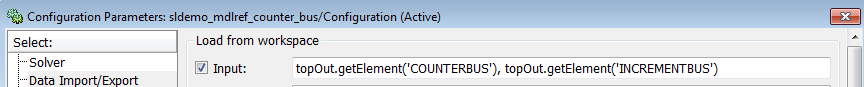## Load Data Logged In Another Simulation

A common source of signal data to load into a model is data that you log from a simulation. You can use the signal data captured from a simulation as roundtrip input to:

• Simulate the same model again from a known starting point.

• Test simulation results.

• Simulate another model starting with the captured signal values from a model. For example, you can log signal data when you simulate a model. Then load the signal data from that simulation as inputs to a second model that you want to reference from the first model.

You can capture the signal data from a simulation in a workspace or in a file. Use one of these techniques to capture signal data from a simulation:

• Signal logging

• To Workspace block

• To File block

• Scope block

• In the Configuration Parameters > Data Import/Export pane, the Output, States, or Final states parameters

• Data store

• The `sim` command configured to log simulation data

For an example of using simulation data for roundtrip signal data loading, see Load Data to Model Variable-Step Input from Model Hierarchy.

Here is a workflow for using signal logging data for standalone simulation of a referenced model. You can use a similar approach for other data logged in `Dataset` format.

1. Use the default signal logging output variable, `logsout`, or specify a variable using the Configuration Parameters > Data Import/Export > Signal logging edit box.

2. Simulate the parent model.

The signal logging output is a `Simulink.SimulationData.Dataset` object.

3. Use the `Simulink.SimulationData.Dataset.getElement` method to access the logged data. The logging data for individual signals is stored in `Simulink.SimulationData.Signal` objects.

4. For the referenced model that you want to simulate standalone, use the `Simulink.SimulationData.Signal.getElement` method to specify signal elements for the Configuration Parameters > Data Import/Export > Input parameter.

For example:5. Simulate the referenced model.

For an example of loading signal logging data for a model that uses model referencing, see Logging Intervals. After you open the model, double-click the blue block labeled Interface Specification and see the sections called:

• Logging Model Reference Signals

Also, the Load Data to Model Variable-Step Input from Model Hierarchy example illustrates loading signal logging data.

To import signal logging data for array of buses signals, see Import Array of Buses Data.

Different logging techniques support different data formats. Most logging techniques support the `Dataset` format, which provides a consistent data format for logged signal data. You can use the `Simulink.SimulationData.Dataset` constructor to convert other data formats to `Dataset` format.